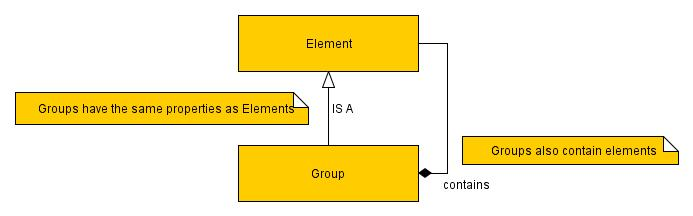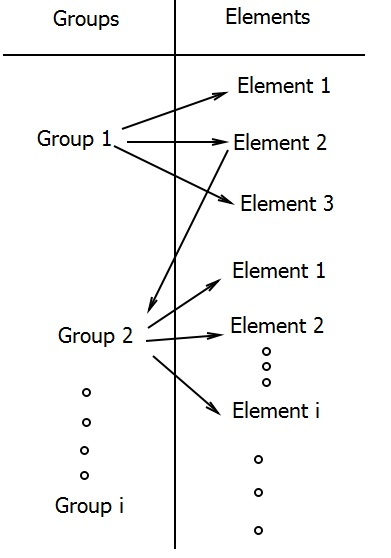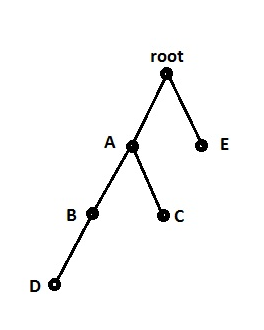# SAGETEA Model and Performance Analysis

Performance Analysis from US patent entitled
“System and Method to Transform a Complex Database
into a Simple, Faster, and Equivalent Database”
Patent #US 20140129514 A1

Researched by
Dr. Maryam Haghighi – University of Ottawa (2014)

SAGETEA Method
Let C be the set of all databases. Let C ⊂ C be the set of all structured query databases, e.g. SQL for relational databases.

Let A represent SAGETEA method, as described in the next section, and let B represent the set of all other methods for structured queries on the set C . We will show that for meaningful queries on finite sets, the worst-case performance of A is better than the worst-case performance of B. A query is order-independent if the ordering of objects in the structure does not affect the results of the query. SAGETEA is constructed for order-independent queries. Define the class called Elements to be the class constructed of the attributes. Define the class called Groups as a subclass of Elements, and thereby inheriting from Elements. Furthermore, instances of Groups contain instances of Elements. We will assume that the number of objects defined as Groups, m, and the number of objects defines as Elements, n, are both finite. See Figure 1 for a representation of Groups and Elements. In SAGETEA implementation, instances of the class Groups are stored in the table GROUPS-TABLE and instances of the class Elements are stored in the table ELEMENT-TABLE. Every instance of Elements is assigned a unique ID.Figure 1: Groups and Elements

We allow for a group to be one of the elements of another group. Thus, we establish a two directional connection between GROUPS-TABLE and ELEMENTS-TABLE, one direction from GROUPS-TABLE to ELEMENTS-TABLE joining an element of each group to its respective group, and the other direction from ELEMENTS-TABLE to GROUPS-TABLE, allowing for a group to be an element of another group. See Figure 2 for an example.Figure 2: Relationship between groups and elements

Consider a schema S as a labelled directed tree, where the nodes represent groups or elements, and the links represent the relationships between the nodes. We assume that every node is a child of another node, except for the root. Define the ancestors of a node v to be all the nodes along the path from the root to the node v. Define the descendants of a node v to be all nodes v 1 , . . . , v k such that v is their ancestor. For example, in Figure 3, node A is an ancestor of nodes C and D, and nodes B,C, and D are descendants of A. The level of a node is defined by letting the 3root be at level one. If a node is at level l, then all of its children are at level l + 1. The depth of a tree is defined as the maximum level of any node in the tree.Figure 3: Tree

Furthermore, in SAGETEA, we assume that the identity of the first group is given by the user once a specific query is made. Once the user requests a query Q with first group G 1 , SAGETEA starts from the respective group G 1 in the GROUPS-TABLE and find all of the elements E 1 , . . . , E k linked to G 1 . If there are no groups among E 1 , . . . , E k , the program returns this set as the output. Otherwise, if there exists an element E i such that E i = G j for some 1 ≤ i ≤ k and 1 ≤ j ≤ m, the algorithm goes along the path (link) from node E i to node G j . Subsequently, the algorithm finds 4all nodes that are linked to G j , and this continues until all of the nodes that are linked in this manner are found.

We assume that throughout this algorithm, if an object has been retrieved once, it will not be retrieved again. That is, if G i , for some i, has already been retrieved as one of the elements of a node in previous steps, it will not be retrieved again as one of the elements of another node, even if it belongs to this second set of elements. This assumption eliminates any possibility of the algorithm to not terminate.

For the purpose of algorithmic analysis, we use the tree representation as defined above. We start by constructing the rooted tree generated from the query Q made by the user. Next, node G 1 corresponding to Q is visited. Afterwards, all descendants of G 1 get visited. The output of the algorithm is the subtree rooted at G 1 . This can be achieved by using any of the common methods for tree traversal. Note that a subtree rooted at a vertex v, is itself another rooted tree. For example, consider Inorder traversal. Inorder traversal consists of moving down the tree toward left until you cannot go any further. Then visit the node, and move one node to the right and continue in the recursive manner.

Note that Inorder traversal can be represented in a non-recursive manner. For a tree of n nodes, it can be shown that the space required for non-recursive inorder traversal is at most n and the time complexity is of O(n) .

Comment: Note that it is sufficient to construct the model using binary trees. This claim is supported by the fact that any k-ary tree can be transformed into a binary tree, using the following method known as ”Left Child-Right Sibling Representation” . To obtain this representation note that every node has at most one leftmost child and at most one closest right sibling. Then, in the binary tree, the left child of a node is the leftmost child (if any) of that node in the k-ary tree, and the right sibling of a node in the binary tree is the closest right sibling (if any) of the corresponding node in the k-ary tree. This method gives a degree 2 representation of any k-ary tree. Note that in such a representation, the right child of the root node of the tree is always empty, since the root has no siblings in the k-ary tree. Thus, any tree can be transformed into a binary tree. Consider a binary tree T . The maximum number of nodes at each level i is 2 i−1 for i ≥ 1. Thus, the maximum number of nodes in a binary tree of depth k is 2 k 1, k ≥ 1. It can be shown that the height of a complete binary tree with n nodes is log 2 (n + 1) .

Comparison to other methods
Standard complexity analysis includes measuring how run time grows as a function of input size. Note that for a database, this means we will use Boolean (yes/no) queries to eliminate depen dence on output size, and the input size is equal to database size plus query size. We use conjunctive queries which are the most common SQL queries (Select-Project-Join). It has been shown that the combined complexity of conjunctive queries is NP-complete . Evaluation of large conjunctive queries is a difficult problem for databases. This is mainly due to the fact that the database is required to evaluate a long sequence of joins and projections. Thus, the query optimizer has to search a very large space and continuously move from one table to another, which may result in great amount of time/space to finish the query.

Using SAGETEA, the need for constructing several tables is eliminated through an efficient construction of only two tables, namely GROUPS-TABLE and ELEMENTS-TABLE. As discussed above, all queries can be found by moving along these two tables, which can also be viewed as a tree traversal. Tree traversal can be accomplished in O(n) which is a great reduction from the typical time and space required by methods using multiple tables.

References
 Horowitz, E., Sahni, S., Mehta, D., Fundamentals of Data Structure in C++, Computer Science Press, New York, 1995.
 Chandra, A.K., Merlin, P.M., Optimal Implementation of Conjunctive Queries in Relational Data Bases, Proceedings of the ninth annual ACM symposium on Theory of computing, 1977.